# Way to Use Lookup formula in Data Validation in Excel

Original Question:-

How to use Lookup formula in Data Validation?

What i want to do sounds simple but I haven't found a solution.

If name/type is "cable" I want a dropdown list for "att" allowing only code1 values.

If name/type is "htrc" I want a dropdown list for "att" allowing only code2 values.

What I've tried is:

- defining a named range called "codes" for \$A:\$A on sheet1

- data validation for B2 on sheet2 and used formula: =IF(\$A2="cable";INDIRECT(codes="code1");INDIRECT(codes="code2"))

When I'm entering that I'm getting a message saying "The Source currently evaluates to an error. Do you want to continue?" and the dropdown is blank…

How to apply

We create the drop down list and we use the “OFFSET”, “MATCH”, “VLOOKUP” and “COUNTIF” in data validation.

• Select the cell B2 in sheet 2.
• Go to Data tab > Data Validation > Settings > List.
• Enter the formula:- =OFFSET(codes, MATCH(VLOOKUP(G6, Keynames, 2, 0), codes, 0)-1, 1, COUNTIF(codes, VLOOKUP(G6, Keynames, 2, 0)), 1)
• Click on ok.
• Copy the Data validation in paste in the range.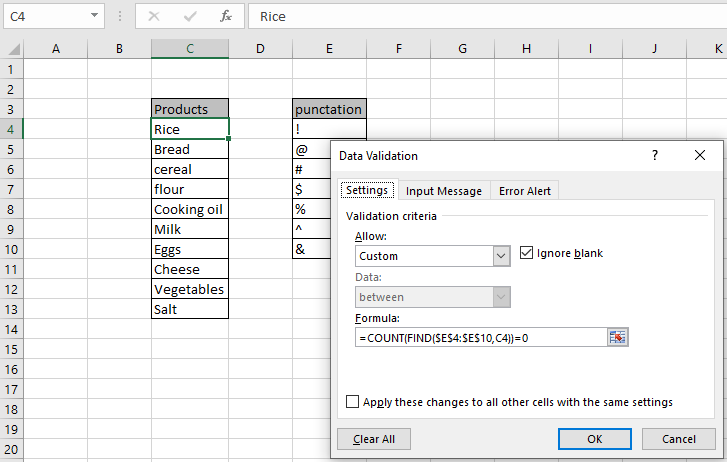Scenario:

Data validation tool restricts the user to input data to ensure its accuracy and consistency in Excel. It helps in the consistency of the data. It guides the user to input the type of option which is necessary for your worksheet. Here the problem is, we need to check the cell, if it has punctuation or not. If the cell has any punctuation mark, that value must be rejected by Excel. If you want to learn more about Data validation in Excel, follow the link. Here we will just consider the problem, allow values only with no punctuation.

How to solve the problem?

Here we have a task that only allows entries with no punctuation. So we need to focus on a list of punctuation to check.

The use of functions and a tool will help us get through the problem.

The formula be used in Data Validation tool. The formula checks the condition and returns True or False. Here the True means that the punctuation doesn't exist, so the tool accepts the value.

Generic formula:

 =COUNT(FIND(pun_list,cell_check))=0

Explanation:

1. FIND function returns the occurrence index of any punctuation in the check cell.
2. COUNT function counts the numbers and matches the result with 0.

Example :

All of these might be confusing to understand. So, let's test this formula via running it on the example shown below. Here we will apply data validation on the cell to restrict the user to input any value which has punctuation. So below is the formula to use in the data validation tool.

Use the formula:

 =COUNT(FIND(\$E4:\$E10,C4))=0

\$ : freezes reference

\$E\$4:\$E\$10 : array of all punctuation

C4 : cell to check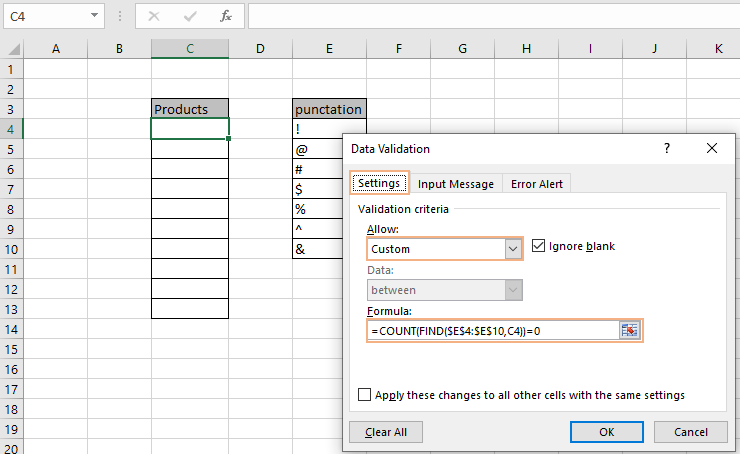Here we have punctuation in the E4:E10 array and to check is C4 cell. Now here the formula is used for the C4 cell. Copy the formula to other remaining cells using the shortcut Ctrl + D. Now we will input values in the cell to check.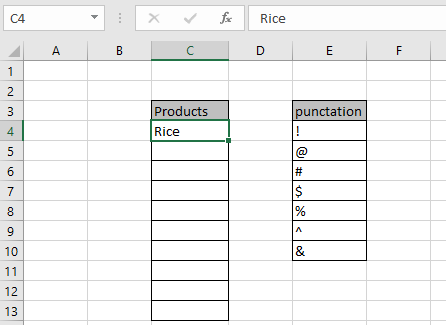Now we use the value with punctuation.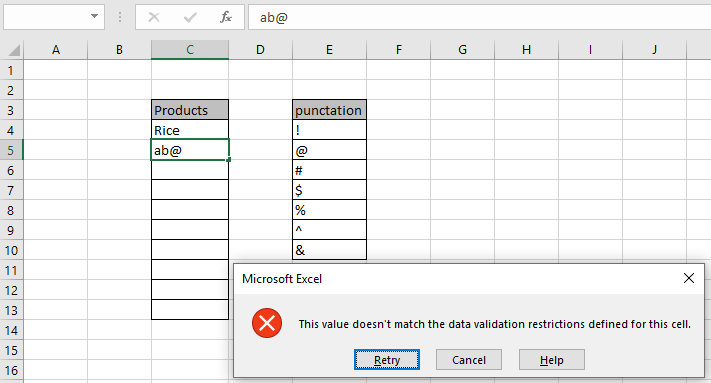As you can see the value is not accepted by Excel and throws an error "This value doesn't match the data validation restrictions defined for this cell". You can also customize the error message and alert message to let users know what values are prohibited. Edit values as shown below.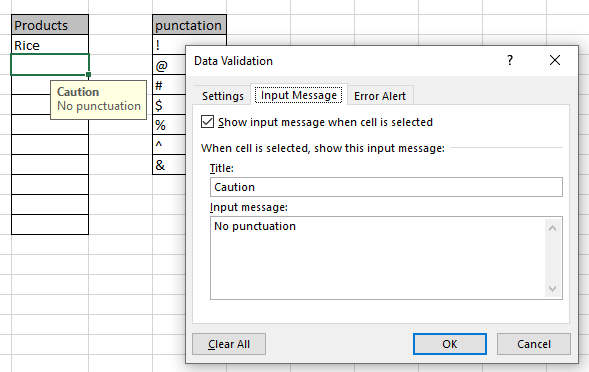As you can see, there is a caution sign saying "No punctuation". Now edit the error message.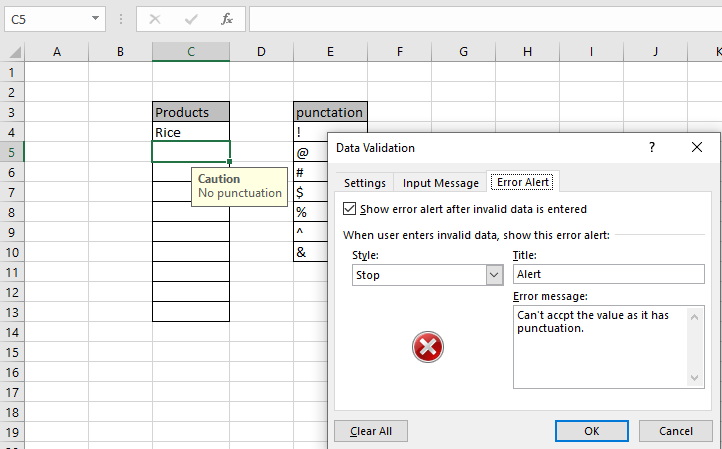As you can see, you get the caution and alert message as required. This Error alert message will be shown whenever a punctuation value is used. Now fill your database without hesitation.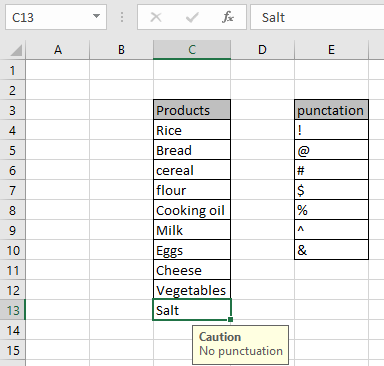As you can see here are all the values without punctuation.

Here are all the observational notes regarding using the formula.

Notes:

1. Apply the data validation tool to restrict the user to input invalid value, which doesn't satisfy the condition.
2. Data validation tool can work with numbers and text both.
3. COUNT and FIND function, both return a number value.
4. Operators like equals to ( = ), less than equal to ( <= ), greater than ( > ) or not equals to ( <> ) can be performed within function applied with numbers only.

Hope this article about Way to Use Lookup formula in Data Validation in Excel is explanatory. Find more articles on Date & Time functions here. If you liked our blogs, share it with your friends on Facebook. And also you can follow us on Twitter and Facebook. We would love to hear from you, do let us know how we can improve, complement or innovate our work and make it better for you. Write us at info@exceltip.com

Related Articles:

Data Validation in Excel : Data Validation is a tool used to restrict users to input value manually in cell or worksheet in Excel. It has a list of options to choose from.

Way to use Vlookup function in Data Validation : Restrict users to allow values from the lookup table using Data validation formula box in Excel. Formula box in data validation allows you to choose the type of restriction required.

Restrict Dates using Data Validation : Restrict user to allow dates from a given range in cell which lays within Excel date format in Excel.

How to give the error messages in Data Validation : Restrict users to customize input information in the worksheet and guide the input information through error messages under data validation in Excel.

Create Drop Down Lists in Excel using Data Validation : Restrict users to allow values from the drop down list using Data validation List option in Excel. List box in data validation allows you to choose the type of restriction required.

Popular Articles :

How to use the IF Function in Excel : The IF statement in Excel checks the condition and returns a specific value if the condition is TRUE or returns another specific value if FALSE.

How to use the VLOOKUP Function in Excel : This is one of the most used and popular functions of excel that is used to lookup value from different ranges and sheets.

How to Use SUMIF Function in Excel : This is another dashboard essential function. This helps you sum up values on specific conditions.

How to use the COUNTIF Function in Excel : Count values with conditions using this amazing function. You don't need to filter your data to count specific values. Countif function is essential to prepare your dashboard.

Terms and Conditions of use

The applications/code on this site are distributed as is and without warranties or liability. In no event shall the owner of the copyrights, or the authors of the applications/code be liable for any loss of profit, any problems or any damage resulting from the use or evaluation of the applications/code.